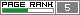## Tuesday, February 27, 2007

### Evolving Lens Through Evolution Strategy And Genetic Algorithm

I used Frank Kursawe's demo applets to evolve lens. In that problem, the goal is to optimize the shape of lens in such a way, that parallel rays falling into the lens will focus in one single point. The shape is optimized by modifying the lens segments' thickness.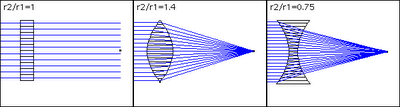In the image above, we have three kinds of lens with a different number of segments. From left to right: 10 segments, 15 segments, and 20 segments. The proportion r2/r1 is the refraction proportion (lens/medium).

Below there is the fitness (or quality) function:di is the distace between the third intersection point and the focal point on the focus plane. Should be noticed that for each incoming ray, there are three intersection points and two refracted rays. The image below illustrates it: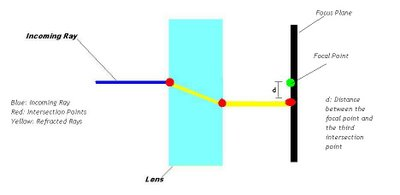It is a minimization problem (smaller the fitness value, better our solution is), since the evolutionary algorithm must find the best (or, at least, a near optimal) set of di's such that diD and D = {d1o, d2o, d3o, ... , dno}, where i ∈ N (natural numbers) and D is the set of distances di's that gives us an optimal or near-optimal solution through minimizing the fitness function parameters, that is, the set D must have the minimal distances between the incidence point of each refracted ray outgoing the lens and the focal point located at the focus plane. In the image above is represented only one refracted ray outgoing the lens, its incidence point (red spot on focus plane) and the distance d between that point and the focal point (green spot). So, that distance d must be minimal for each ray, because by this way we can minimize the fitness function.

SIMULATIONS:

EVOLUTION STRATEGY CONFIGURATION:

Number Of Parents: μ = 5
Number Of Offsprings: λ = 35
Selection Scheme: Comma Selection*
Mutation: Yes
Recombination: No (ρ = 0)
Step-Size Value (α): α = 2.05

*When we use the comma selection (notation (μ, λ)-ES), we are saying that μ parents will generate λ offsprings from which the top μ will be selected to become parents in the next generation, although the top μ are worse than the previous parents, that is, the offspring's fitness is worse than the parents'. So, using μ = 5 and λ = 35, we are saying that five parents will generate 35 offsprings from which the best five ones will be selected to be parents in the next generation, even if those five ones are worse than the parents that they are replacing. It helps to escape from local optima and to track a moving optimum. That kind of selection scheme may alow a temporary degradation of the best current individual inside the population, but, at same time, that mechanism tries to keep the search in a path toward the (near) optimum. This optimum seeking is achieved, also, through self-adaptation, since there are other factors whose can influence the optimum tracking, such as population size.

Using the notation we have a (5, 35)-ES.

GENETIC ALGORITHM CONFIGURATION:

Population Size: 400
Selection Scheme: Roulette Wheel
Recombination Rate (pc): pc = 0.8
Mutation Rate (pm): pm = 0.01
Recombination Type: Two point
Mutation Type: Bit inversion
Elitism: No

As we do not have elitism, our Genetic Algorithm can be considered the so-called Canonical (or Simple) Genetic Algorithm (CGA or SGA).

RESULTS:

Evolution Strategy:

Number Of Generations: 363
Best Fitness Function Value Found: 0.9865042138018424

Genetic Algorithm:

Number Of Generations: 9000
Best Fitness Function Value Found: 28.921995890607977

Now, let's use recombination and set α with the recommended value of 1.3 (Rechenberg) to the evolution strategy. For the genetic algorithm, let's apply elitism to speed up the search. So, the new configurations are as follow:

EVOLUTION STRATEGY CONFIGURATION:

Number Of Parents: μ = 5
Number Of Offsprings: λ = 35
Selection Scheme: Comma Selection
Mutation: Yes
Recombination: Yes (ρ = 2)
Recombination Type: Discrete
Step-Size Value (α): α = 1.3 (Rechenberg)

GENETIC ALGORITHM CONFIGURATION:

Population Size: 400
Selection Scheme: Roulette Wheel
Recombination Rate (pc): pc = 0.8
Mutation Rate (pm): pm = 0.01
Recombination Type: Two point
Mutation Type: Bit inversion
Elitism: Yes
Elite-Size Value: 1

When we apply elitism, we are keeping the best solution found so far inside the population. It does not mean that solution is the optimum, it is only the best result got by the search until the current generation. The use of elitism has the objective to speed up the search, that is, to converge faster toward the optimum. However, there are some arguments which criticize that kind of strategy, since it can prematurely trap the search in local optima.

RESULTS:

Evolution Strategy:

Number Of Generations: 104
Best Fitness Function Value Found: 0.6014123658591052

Genetic Algorithm:

Number Of Generations: 7000
Best Fitness Function Value Found: 10.065706063553371

The evolution strategy found, again, better solutions and needed less generations to reach an (sub-)optimal solution. Surely it has to do with the use of recombination, since the theory says that evolution strategies which have recombination mechanisms are less prone to get stuck in local optima and can accelerate the convergence rate toward an optimum. However, would be very sensible to remember that the recombination theory from evolution strategies holds mainly if we consider (μ/μI/D, λ)-ES, that is, we have ρ = μ, what means we can have a number μ of parents involved in the production of just one offspring. When we have 1 < ρ < μ, then, the evolution strategy can behave between those two extrema for ρ.

Below we have the progress rate (φ*) formulae for the (μ, λ)-ES
(notation φ*μ, λ) and the (μ/μD, λ)-ES (φ*μ/μD, λ), respectively: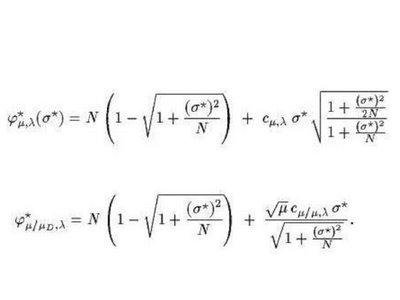It is easier to understand those formulae through a simplified form made by Ingo Rechenberg, see below: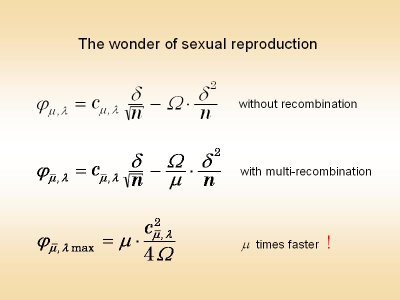It's clear that the Multi-Recombinative Evolution Strategy has a coeficient progress (φ) which is μ times faster than the Non-Recombinative Evolution Strategy. Then, the former has a convergence speed larger than its version without multi-recombination.

The Genetic Algorithm failed to find a reasonable solution for the problem, while the Evolution Strategies, both Recombinative and Non-Recombinative, got (near) optimal solutions. The explanation for that could be the fact that Evolution Strategies have self-adaptation mechanisms that allow them to be less susceptible to get trapped in local optima and the recombination scheme is able to shrink the "negative" effects of mutation, what is interesting because it can guide the search through a path toward the (near) optimum solution, that is, the Evolution Strategy has a more robust mechanism to maintain an optimum seeking.

The Genetic Algorithm has no self-adaptation mechanisms and the search relies only in the probabilities associated with the evolutionary operators (Selection + Crossover + Mutation), that is, each individual inside the current population has a probability to be selected and those which are selected can or cannot be subject to crossover and/or mutation. Those probabilities can generate difficulties to exchange genetic information among strings, because, even though some fit strings are selected to crossover and/or mutation, we may miss the chance to combine strings which have important genetic information that could guide better the search and, sometimes, it is necessary to modify all the variables at same time to improve the search (escape local optima), what is an unlikely event due to those probabilities associated to each evolutionary operator. This can trap the search in local optima and even obstruct all the search process. The crossover operator can disrupt good individuals (bit strings with nice fitnesses) and generate an offspring which is not so interesting as its parents were. Even short, low order, above average schemata can be broken into schemata that are not interesting and, worse, can put the search in a path far away from optimum. The mutation operator, as it has very small probability rate, is called rarely if compared to crossover operator and the degree of new bits put inside the population is very low, this can be harmful because the crossover operator is called so many times (remember that crossover has large probability rate) and the innovation power of crossover is limited, since it can only work upon genetic information already in the population.

Another reason for the poor performance of the Genetic Algorithm, would be the fact that the fitness function above is not separable/decomposable. Some investigations already explained that the Genetic Algorithm's evolutionary operators (crossover and mutation) and the probabilities associated with each one is an interesting strategy to find optimal solutions in decomposable/separable functions. Below there is what is a decomposable/separable function:

Consider the hyperplane function:

F(x1, x2, ..., xn) = x1 + x2 + ... + xn. (1)

That function can be optimized as n independent optimizations:

opt[F(x1, x2, ..., xn)] = opt[x1] + opt[x2] + ... + opt[xn]. (2)

So,

opt[F(x1, x2, ... xn)] = ∑i opt[fi(xi)], (3)

where 1 ≤ i ≤ n and i is a natural number in the set N*. If (3) is satisfied, then the function is decomposable/separable.

However, I guess that the fitness function above is decomposable.

Let's be fair: We have nowadays new generation Genetic Algorithms, the so-called Estimation of Distribution Algorithms or EDAs, whose can deal, at some extent, with non-decomposable/non-separable functions. Some of those EDAs assume a degree of interdependency among fitness function's variables, such as bivariate dependencies and multivariate dependencies, and others EDAs can only deal with problems which have full independency among their variables (Compact Genetic Algorithm - cGA; Univariate Marginal Distribution Algorithm - UMDA; Population Based Incremental Learning - PBIL). In EDAs there is a trade off between the accuracy of the estimation and its computational cost, that is, more precisely the estimation is, more computationally expensive it is to calculate.

## Friday, February 23, 2007

### See You, Mr. Fogel...I have a sad news to give you: I knew from IlliGAL Blogging that Dr. Lawrence Fogel, a pioneer inside evolutionary computation field and one of the founders of evolutionary programming, passed away on Sunday due to renal failure. It's a sad day to evolutionary computation and to us all.RIP

## Thursday, February 08, 2007

### ROUTINE ORDER Blogs About HEC

ROUTINE ORDER wrote (see here) about the post I made upon the History Of Evolutionary Computation (HEC), the so-called Evolutionary Computation Classics - Volume I which deals with the early steps of Evolution Strategies.

Thank you, friend, for your nice words! :)

I will make some improvements in the text of the "Evolutionary Computation Classics - Volume I" and, also, write about CMA-ES and other things I forgot.

Surely that there will be "Evolutionary Computation Classics" about Genetic Algorithms and Evolutionary Programming too. :)